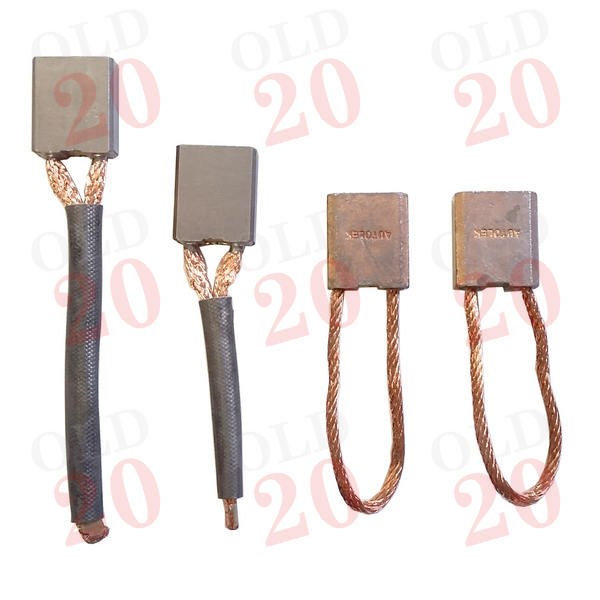View larger

# Starter Motor Brush Set

2062601

New

£ 7.20Tractors < Case IH < 94 < 1194
Tractors < Case IH < 94 < 1294
Tractors < Case IH < 94 < 1394
Tractors < Case IH < 94 < 1494
Tractors < County < Various < 654
Tractors < County < Various < 754
Tractors < County < Various < 764
Tractors < County < Various < 774
Tractors < County < Various < 944
Tractors < County < Various < 964
Tractors < County < Various < 974
Tractors < David Brown < 700 < 770
Tractors < David Brown < 700 < 780
Tractors < David Brown < 800 < 880 (Selectamatic)
Tractors < David Brown < 800 < 885
Tractors < David Brown < 900 < 990 (Selectamatic)
Tractors < David Brown < 900 < 995
Tractors < David Brown < 900 < 996
Tractors < David Brown < 1200 < 1200
Tractors < David Brown < 1200 < 1210
Tractors < David Brown < 1200 < 1212
Tractors < David Brown < 94 < 1294
Tractors < David Brown < 94 < 1394
Tractors < David Brown < 94 < 1494
Tractors < Tractors < Ferguson < TEF20
Tractors < Tractors < Ferguson < FE35 (23c)
Tractors < Fordson and Ford < Vintage < E1A Major
Tractors < Fordson and Ford < Vintage < Power Major
Tractors < Fordson and Ford < Vintage < Super Major
Tractors < Fordson and Ford < Vintage < Dexta
Tractors < Fordson and Ford < Vintage < Super Dexta
Tractors < Fordson and Ford < 1000 Series < 2000
Tractors < Fordson and Ford < 1000 Series < 3000
Tractors < Fordson and Ford < 1000 Series < 4000
Tractors < Fordson and Ford < 1000 Series < 5000
Tractors < Fordson and Ford < 1000 Series < 7000
Tractors < Fordson and Ford < 100 Series < 2600
Tractors < Fordson and Ford < 100 Series < 3600
Tractors < Fordson and Ford < 100 Series < 3900
Tractors < Fordson and Ford < 100 Series < 4100
Tractors < Fordson and Ford < 100 Series < 4600
Tractors < Fordson and Ford < 100 Series < 5600
Tractors < Fordson and Ford < 100 Series < 5700
Tractors < Fordson and Ford < 100 Series < 6600
Tractors < Fordson and Ford < 100 Series < 6700
Tractors < Fordson and Ford < 100 Series < 7600
Tractors < Fordson and Ford < 100 Series < 7700
Tractors < Landini < 60 < 5860
Tractors < Landini < 60 < 6060
Tractors < Landini < 60 < 6860
Tractors < Landini < 60 < 7860
Tractors < Landini < 60 < 8860
Tractors < Landini < 80 < 6880
Tractors < Landini < 80 < 7880
Tractors < Landini < 80 < 8880
Tractors < Leyland Marshall < Leyland < 245
Tractors < Leyland Marshall < Leyland < 253
Tractors < Leyland Marshall < Leyland < 342
Tractors < Leyland Marshall < Marshall < 502
Tractors < Massey Ferguson < Pre-100 Series < 35
Tractors < Massey Ferguson < Pre-100 Series < 35X
Tractors < Massey Ferguson < Pre-100 Series < 65
Tractors < Massey Ferguson < 100 < 134V
Tractors < Massey Ferguson < 100 < 148
Tractors < Massey Ferguson < 100 < 168
Tractors < Massey Ferguson < 100 < 175
Tractors < Massey Ferguson < 100 < 178
Tractors < Massey Ferguson < 100 < 185
Tractors < Massey Ferguson < 100 < 188
Tractors < Massey Ferguson < 200 < 230
Tractors < Massey Ferguson < 200 < 240
Tractors < Massey Ferguson < 200 < 250
Tractors < Massey Ferguson < 200 < 255
Tractors < Massey Ferguson < 200 < 265
Tractors < Massey Ferguson < 200 < 275
Tractors < Massey Ferguson < 200 < 285
Tractors < Massey Ferguson < 200 < 290
Tractors < Massey Ferguson < 300 < 350
Tractors < Massey Ferguson < 300 < 353
Tractors < Massey Ferguson < 300 < 355
Tractors < Massey Ferguson < 300 < 360
Tractors < Massey Ferguson < 300 < 363
Tractors < Massey Ferguson < 300 < 373
Tractors < Massey Ferguson < 300 < 377
Tractors < Massey Ferguson < 300 < 383
Tractors < Massey Ferguson < 300 < 387
Tractors < Massey Ferguson < 300 < 390
Tractors < Massey Ferguson < 300 < 393
Tractors < Massey Ferguson < 300 < 397
Tractors < Massey Ferguson < 500 < 550
Tractors < Massey Ferguson < 500 < 565
Tractors < Massey Ferguson < 500 < 575
Tractors < Massey Ferguson < 500 < 590
Tractors < Massey Ferguson < 600 < 690
Tractors < Massey Ferguson < 3000 < 3060
Tractors < Tractors < Nuffield < 10 < 42
Tractors < Tractors < Nuffield < 10 < 60
Tractors < Tractors < Nuffield < 3 < 45
Tractors < Tractors < Nuffield < 3DL
Tractors < Tractors < Nuffield < 4 < 60
Tractors < Tractors < Nuffield < 4 < 65
Tractors < Tractors < Nuffield < 4DM
Tractors < David Brown < 90 < 1190
Tractors < David Brown < 90 < 1290
Tractors < David Brown < 90 < 1390
Tractors < David Brown < 90 < 1490
Tractors < David Brown < 94 < 1194

Supplier Reference :GSB101, 705833R91, 37H5030, MBU1222, 1012013M91, K962799, 81829254, D2NN11055A

 Section Electrical